# Multiple Choice Questions and Answers on Analog Electronics

Q1. Which of the following oscillators is suitable for frequencies in the range of mega hertz?

1. RC phase shift
2. Wien bridge
3. Hartley
4. Both (1) and (3)

Explanation: Only LC oscillators are suitable for MHz range

Q2. For a base current of 10 μA, what is the value of collector current in common emitter if βdc = 100

1. 10 μA
2. 100 μA
3. 10 mA
4. 1 mA

Explanation: IC = 10 x 100 μA = 1 mA

Q3. To prevent a DC return between source and load, it is necessary to use ………………

1. resistor between source and load
2. inductor between source and load
3. capacitor between source and load
4. either (1) or (2)

Explanation: Capacitor offers infinite impedance to DC

Q4. A half wave diode circuit using an ideal diode has an input voltage 20 sin ωt volts. Then average and rms values of output voltage are ………… and ……………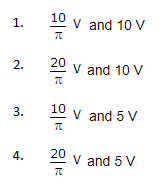Explanation:Q5. If the input to the ideal comparator shown in the figure is a sinusoidal signal of 8 V (peak to peak) without any DC component, then the output of the comparator has a duty cycle of …………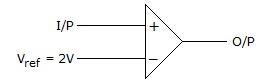1. 1/2
2. 1/3
3. 1/6
4. 1/12

Explanation: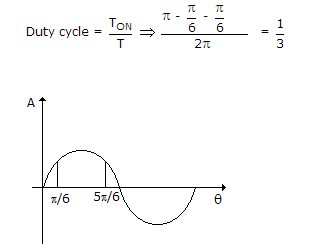Q6. If an amplifier with a gain of – 1000 and feedback factor β = – 0.1 had a gain change of 20% due to temperature, the change in the gain of the feedback amplifier would be …………….

1. 10%
2. 5%
3. 0.2%
4. 0.01%

Explanation: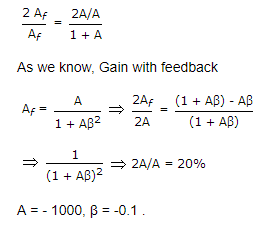Q7. The load impedance ZL of a CE amplifier has R and L in series. The phase difference between output and input will be ………….

1. 180°
2. 0
3. more than 90° but less than 180°
4. more than 180° but less than 270°

Explanation: It is 180° for purely resistive load and between 180° and 270° for R-L load

Q8. An RC coupled amplifier has an open loop gain of 200 and a lower cutoff frequency of 50 Hz. If negative feedback with β = 0.1 is used, the lower cut off frequency will be ……………

Explanation: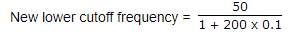Q9. Gain of the amplifier is ‘A’. Then the I/P impedance and O/P impedance of the closed loop amplifier shown below would be …………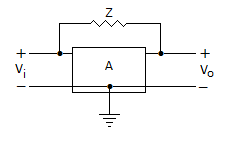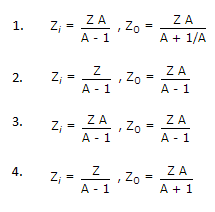Explanation: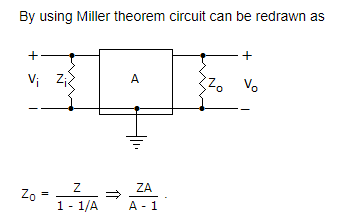Q10. In the figure below v1 = 8 V and v2 = 4 V. Which diode will conduct?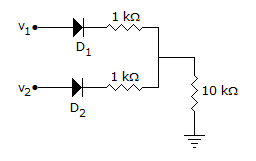1. D2 only
2. D1 only
3. Both D1 and D2
4. Neither D1 nor D2

Explanation:

D1 will conduct and the output voltage will be about 7 V. Therefore D2 will be reverse biased and will not conduct.

Q11. The action of JFET in its equivalent circuit can best be represented as a

1. current controlled current source
2. current controlled voltage source
3. voltage controlled voltage source
4. voltage controlled current source

Q12. In a CB amplifier the maximum efficiency could be

1. 99%
2. 85%
3. 50%
4. 25%

Q13. In a pn junction diode under reverse bias, the magnitude of electric field is maximum at

1. the edge of the depletion region on the p side
2. the edge of the depletion region on the n side
3. the pn junction
4. the center of the depletion region on the n side

Q14. To prevent a DC return between source and load , it is necessary to use  ………….

1. resistor between source and load
2. inductor between source and load
3. capacitor between source and load
4. both 1 and 2

Q15. The cascade amplifier is a multistage configuration of ………….

1. CC-CB
2. CE-CB
3. CB-CC
4. CE-CC

Q16. which of the following statement is true ?

Negative feedback in an amplifier

1. reduces gain
2. increase frequency and phase distortion
3. reduces bandwidth
4. increases noise

Q17. The most commonly used amplifier in sample and hold circuit is ……………

1. a unity gain non-inverting amplifier
2. a unity gain inverting amplifier
3. an inverting amplifier with a gain of 10
4. an inverting amplifier with a gain of 100

Q18. In a common-emitter, unbypassed resistor provides ………….

1. voltage shunt feedback
2. current series feedback
3. negative voltage feedback
4. positive current feedback

Q19. The current gain of a bipolar transistor drops at high frequencies because of …………..

1. Transistor capacitances
2. High current effects in the base
3. Parasitic inductive elements
4. the early effect

Q20. Generally, the gain of a transistor amplifier falls at high frequencies due to …………….

1. Internal capacitance of the device
2. Coupling capacitor at the input
3. Skin effect
4. Coupling capacitor at the output

Q21. Voltage series feedback results in ……………

1. Increase in both input and output impedances
2. Decreases in both input and output impedances
3. Increase in input impedance and decreases in output impedance
4. Decreases in input impedance and increase in output impedance

Q22. MOSFET can be used as  a …………

1. Current controlled capacitor
2. Volatge controlled capacitor
3. Current controlled inductor
4. Voltage controlled induact

Q23. A change in the value of the emitter resistance Re in a differential amplifier

1. Affects the difference mode gain Ad
2. Affects the common mode gain Ac
3. Both 1 and 2
4. Does not affect either Ad or Ac

Q24. The bandwidth of an RF tuned amplifier is dependent on ………..

1. Q-factor of the tuned o/p circuit
2. Q-factor of the tuned i/p circuit
3. Q-Point
4. Q-factor of the o/p and i/p circuits as well as Q-point

Q25. The MOSFET switch in its ON state is equivalent to ……………

1. Resistor
2. Inductor
3. Capacitor
4. Battery

Q26. Most of the linear ICs are based on the two-transistor differential amplifer because of its ……….

1. Input voltage-dependent linear transfer characteristics
2. High voltage gain
3. High input resistance
4. High CMRR

Q27. The horizontal intercept of a DC load line is the same as ideal ………….

1. Cut-off point
2. Satuaration point
3. Operating point
4. Quasi-saturation point

Q28. A differential amplifier is invariably used in the i/p stage of all OP-Amps . This is done basically to provide the OP-Amps with a very high …………….

1. CMRR
2.  Bandwidth
3. Slew rate
4. Open-loop gain

Q29. The early effect in a BJT is caused by ………….

1. Fast turn-on
2. Fast turn-off
3. Large collector-base reverse bias
4. Large emitter-base forward bias

Q30. In a transistor leakage current mainly depends on …………….

1. Doping of base
2. size of emitter
3. rating of the transistor
4. Temperature

Q31. A source follower using a FET usually has a voltage gain which is ………….

1. Greater than 100
2. Slightly less than 1 but positive
3. Exactly unity but negative

Q32. In an OP-amp differentiator

1. The amplitude of output is proportional to rate of change of input
2. The amplitude of output is proportional to input
3. Output occurs when input is finite and constant
4. Polarity of input and output is the same

Q34. Class AB operation is often used in power large signal amplifies in order to ……………..

1. Get maximum efficiency
2. Remove even harmonics
3. Overcome a crossover distortion
4. Reducing collector dissipation

Q35. Which of the following amplifier circuit using junction transistor has the best gain?

1. Common base
2. Common emitter
3. Common collector
4. All have the same gain

Q36. When a transistor is connected in common emitter mode, it will have …………………

1. Negligible input resistance and high output resistance
2. High input resistance and low output resistance
3. Medium input resistance and high output resistance
4. Low input resistance as well as output resistance

Q37. In all base driver amplifiers, …………………..

1. ac collector voltage is 1800 out of phase with ac base
2. ac emitter voltage is 1800 out of phase with ac base voltage
3. ac collector voltage is in phase with ac base voltage
4. none of the above

Q38. The effective channel length of a MOSFET in  saturation, decreases with increase in ………………

1. Gate voltage
2. Drain voltage
3. Source voltage
4. Body voltage

Q39. Which of the following statement is not true?

1. Push-pull signals are equal and opposite in phase
2. class AB operation cannot be used for a push-pull audio power output phase
3. A push-pull output transformer has a center-tapped primary
4. All of the above

Q40. The encapsulation of a transistor is necessary for ……….

2. Preventing photo-emission effect
3. Avoiding loss of free electrons
4. Mechanical ruggedness

Q41. In deriving ac equivalent circuit for an amplifier circuit we short circuit ………….

1. all transistor
2. all resistor
3. all inductors
4. all capacitors